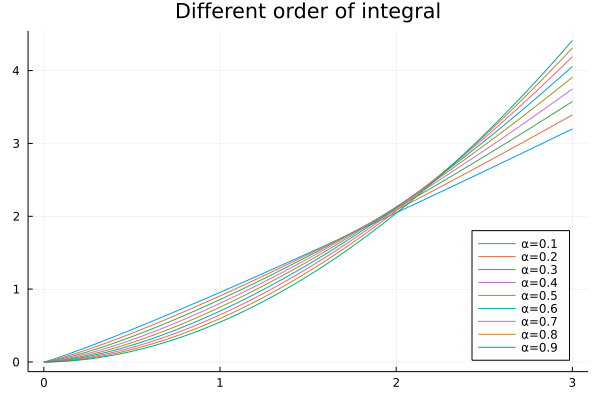# Integral Example

Let's see how easy it is to compute the fractional integral of a function🕺

using FractionalCalculus
using Plots

tspan = collect(0:0.01:3)

exampleintegral = []

for i in range(0.1, 0.9, step=0.1)
push!(exampleintegral, fracint(x->x, i, tspan, 0.001, RLInt_Approx()))
end

plot(tspan, exampleintegral, title="Different order of integral", linewidth=1, label=["α=0.1" "α=0.2" "α=0.3" "α=0.4" "α=0.5" "α=0.6" "α=0.7" "α=0.8" "α=0.9" "α=1"], legend=:bottomright)

Here, we use Plots.jl to generate the computing result: